SCERT AP 7th Class Maths Solutions Pdf Chapter 8 Exponents and Powers Ex 8.1 Textbook Exercise Questions and Answers.

## AP State Syllabus 7th Class Maths Solutions 8th Lesson Exponents and Powers Ex 8.1

Questions 1.
Express the following into exponential form:.
(i) 14 × 14 × 14
14 × 14 × 14 = 143

(ii) 25 × 25 × 25 × 25 × 25
25 × 25 × 25 × 25 × 25 = 25

(iii) ab × ab × ab × ab
ab × ab × ab × ab = (ab)4

(iv) 7 × p × p × q
7 × p × p × q = 7 × p2 × qQuestion 2.
Express the following into expanded form:
(i) 276
276 = 27 × 27 × 27 × 27 × 27 × 27

(ii) 1015
1015 = 101 × 101 × 101 × 101 × 101

(iii) (2b)4
2b4 = 2b × 2b × 2b × 2b

(iv) 3a8
3a8 = 3 × a× a × a × a × a × a × a × a

Question 3.
Express the following In exponential form through prime factorisation: .
(i) 81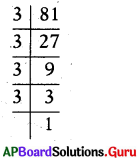81 = 3 × 27
= 3 × 3 × 9
= 3 × 3 × 3 × 3
∴ 81 = 34

(ii) 125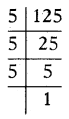125 = 5 × 25
= 5 × 5 × 5
∴ 125 = 53

(iii) 324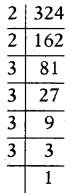324 = 2 × 162
= 2 × 2 × 81
= 2 × 2 × 3 × 11
= 2 × 2 × 3 × 3 × 9
= 2 × 2 × 3 × 3 × 3 × 3
∴ 324 = 22 × 34

(iv) 1080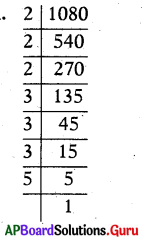1080 = 2 × 540
= 2 × 2 × 270
= 2 × 2 × 2 × 135
= 2 × 2 × 2 × 3 × 45
= 2 × 2 × 2 × 3 × 3 × 15
= 2 × 2 × 2 × 3 × 3 × 3 × 5
∴ 1080 = 23 × 31 × 51Question 4.
Compute and identify the greater num-ber in the following pairs : 7
(i) 25 or 52
25 = 2 × 2 × 2 × 2 × 2 = 32
52 = 5 × 5 = 25
32 > 25
Therefore, 25 > 52

(ii) 73 or 37
73 = 7 × 7 × 7 = 343
37 = 3 × 3 × 3 × 3 × 3 × 3 × 3 = 2187
2187 > 343
Therefore, 37 > 73

(iii) 23 or 32
23 = 2 × 2 × 2 = 8
32 = 3 × 3 = 9
9 > 8
Therefore, 32 > 23

Question 5.
Expand 33 × 42 and 43 × 32. Are they equal? Justify.
33 × 42 = 3 × 3 × 3 × 4 × 4
= 27 × 16 = 432

43 × 32 = 4 × 4 × 4 × 3 × 3
= 64 × 9 = 576

432 × 576 (They are not equal)
Therefore, 576 > 432
So, 43 × 32 > 33 × 42

Question 6.
Express the following numbers in exponential form with the given base.
(i) 1000, base 10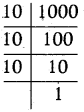∴ 1000 = 10 × 10 × 10 = 103

(ii) 512 base 2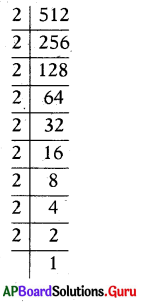512 = 2 × 2 × 2 × 2 × 2 × 2 × 2 × 2 × 2
∴ 512 = 29

(iii) 243 base 3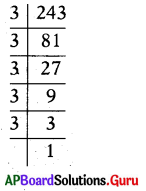243 = 3 × 3 × 3 × 3 × 3
∴ 243 = 35Question 7.
If a = 2, b = 3 find the value of
(i) aa + bb
Given a = 2, b = 3
aa + bb = 22 + 33
= (2 × 2) + (3 × 3 × 3)
= 4 + 27 = 31
Therefore, if a = 2, b = 3 then aa + bb = 31

(ii) ab + ba
Given a = 2, b = 3
ab + ba = 23 + 32
= (2 × 2 × 2) + (3 × 3).
= 8 + 9 = 17
Therefore, if a = 2, b = 3
then ab + ba = 17

(iii) (a + b)b
Given a = 2, b = 3
(a + b)b = (2 + 3)3 = 53
= 5 × 5 × 5 = 125
Therefore, if a = 2, b == 3
then (a + b)b =125

Question 8.
Write the following in Exponential form:
(i) The speed of light in vacuum is about 30,00,00,000 m/sec.